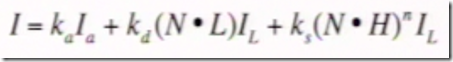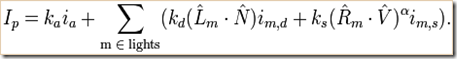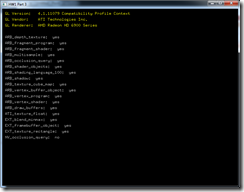This wordpress theme is downloaded from wordpress themes website.

## 3. Transformations I: Linear Transformations (vector, matrix)

3. Transformations I: Linear Transformations. Multiple Transformations (2/14) … vectors & linear transformations (4×4 matrices)

Vector is length & direction, can be represented as 2D (dx, dy) or 3D (dx, dy, dz).  Add vectors adds components (visually attach tail to head).  Subtract vector just add the negative (visually flip direction of minus vector).  To compute the length, use pythagorean theorem.  Unit vector is vector length 1, compute by dividing the vector by its length.

Dot product A dot B = AxBx + AyBy + AzBz = cos(theta) * ||A|| * ||B||.  Theta is the angle between A and B.  ||A|| is magnitude of A.  For unit vectors, A dot B = cos(theta).  Because for unit vectors, ||A|| is 1 and ||B|| is 1.  Dot product can tell you which side of a surface something is on.  Example take the dot product of the Normal vector (to a plane) and the View vector (to the camera); the sign (greater than or less than zero) tells you if the angle is less than or greater than 90 degrees…  Using dot product (instead of a trig function such as cosine).

Cross product in 3D: A cross B gives a third vector perpendicular to A and B.  Use right-hand rule (A pointer finger, B middle, AxB thumb) for right-handed coordinate system.  A cross B = (AyBz – AzBy, AzBx – AxBz, AxBy -  AyBx).  Mnemonic: to memorize this (for fun?), I think of each component’s subscripts for A*B-A*B as going xyzxyz-(reverse of that) and you start at X for X but there’s no X in the X (same for Y & Z).  Cross product gives normal to a plane (used for lighting calculations) (typically 3D models created by 3D modeling software will have pre-computed normals saved as parameters for each vertex).

Linear transformation between two vector spaces is a map such that: L(k*A) = k*L(A) for scalar k.  L(A+B) = L(A) + L(B).  Lines are preserved.  We do linear transformations with matrices.  For 3D graphics, we use 4×4 matrices.  Today we will talk about the top three rows (not the bottom row).  Identity matrix:
(1 0 0 0)(x)   (x)
(0 1 0 0)(y) = (y)
(0 0 1 0)(z)   (z)
(0 0 0 1)(1)   (1)

Scaling (negative values causes reflection).  Translation (not a linear transformation in 3D, but it is in 4D, so we use the 4th column, which is why we have a 4×4 instead of 3×3).  Shearing (stretching, parallel lines stay parallel) by axis (eg, to shear along Z, X and Y values are altered by an amount proportional to the value of Z, without changing Z).
(Sx  Exy Exz  Tx)(x)   (Sx*x + Tx + y*Exy + z*Exz)
(Eyx Sy  Eyz  Ty)(y) = (Sy*y + Ty + x*Eyx + z*Eyz)
(Ezx Ezy Sz   Tz)(z)   (Sz*z + Tz + x*Ezx + y*Ezy)
(0   0   0    1 )(1)   (1)

Rotation about x-axis, using right-hand rule:
(   1      0       0      0   )(x)   ()
(   0    cos(a) -sin(a)   0   )(y) = ()
(   0    sin(a)  cos(a)   0   )(z)   ()
(   0      0       0      1   )(1)   ()

Rotation about y-axis:
( cos(b)   0     sin(b)   0   )(x)   ()

(   0      1       0      0   )(y) = ()
(-sin(b)   0     cos(a)   0   )(z)   ()
(   0      0       0      1   )(1)   ()

Rotation about z-axis:

( cos(c) -sin(c)   0      0   )(x)   ()

( sin(c)  cos(c)   0      0   )(y) = ()
(   0      0       1      0   )(z)   ()
(   0      0       0      1   )(1)   ()

There are other rotations, such as the general case is rotation about an arbitrary line, which can be simplified assuming the arbitrary line is a unit vector.

A taxonomy of some linear transformations.  Rigid Body (preserve distance): rotation, translation.  Similarity (preserve angles): rigid body, uniform scaling / reflection.  Affine (preserve parallel lines): similarity, shear.  Projective (preserves lines => lines stay straight => it’s a linear transformation).

The order of your transformations matters.  For example, if you do rotation then translation, it’s different than if you do translation then rotation.

## 2. Light, Illumination & Reflectance

Notes on lecture, 2. Illumination. What is light? The BRDF. Vectors and dot products. The Phong BRDF model (2/7) … Light-Material interactions, what is light, illumination & reflectance, phong reflectance model, vectors & dot products. … more details about vectors & dot product in lecture 3

Diffuse reflections (incident ray reflects at many angles).  Specular reflections (mirror-like, incident ray reflects at many angles).  Specular highlights (bright spot on shiny object).  Refraction (bends light).  Dispersion (e.g. rainbow) (phase velocity of wave depends on its frequency) (different refractive index for different wavelengths).  Rainbow photo shows refraction, internal reflection, dispersion.  Atmospheric effects (haze, fog, crepuscular rays / god rays, light under water).  Interference (e.g. soap bubbles, thin layer of oil on water) (two waves superimpose to form a resultant wave of greater or lower amplitude).  Diffraction (e.g. CD discs) (physically similar to interference, but diffraction tends to mean lots of interference) (wave encounters obstacle) (disc example, tiny grooves are close to the wavelength of light so it causes some of the light to reflect in phase and some out of phase).  Motion blur.

What is light, wave-particle duality, but basic CG does geometric view of optics (rays of photos), and we simulate wave behaviors as effects.  Newton dispersion.

Human eye detects light (biology).  Visible spectrum of electromagnetic waves is about 390 to 700nm.  Human eye photoreceptor cells: rods adapt to brightness (helps vision in low light), cones detect red & green & blue (trichromatic vision) (cone peaks are short 420-440 nm, middle 530-540 nm, long 560-580 nm).  Metamers (different combinations of light across all wavelengths can produce the same visible color in terms of human eye receptor response).  Humans see light as additive colors (R+G=Yellow, R+B=Magenta, G+B=Cyan, R+G+B=White).  Ink (CMYK printing) & paint & crayons uses subtractive colors (Magenta+Yellow=R, Cyan+Yellow=G, Cyan+Magenta=B, Magenta+Yellow+Cyan=Black).

Illumination light sources: emittance spectrum (color), geometry (position & direction), directional attenuation (falloff).  Illumination surface properties: reflectance spectrum (color), geometry (position, orientation, micro-structure), absorption.

Graphics jargon (he’s using): illumination is transport of energy from light sources between points (direct & indirect paths).  Lighting is compute light intensity reflected from a specific 3D point.  Shading is assign color to a pixel based on illumination in the scene.

Direct illumination: surface point receives light directly from all light sources in scene – determine which light sources are visible, compute by local illumination model; OpenGL.  Global illumination: surface point receives light after light rays interact with other objects; indirect illumination; slow, ray tracing.

Light sources.  Directional light source.  Point light source.  Other: spotlights, area light sources, extended light sources (spherical).

Light is linear – you can add light sources and components.

Reflectance models.  BRDF (bi-directional reflection distribution function).  Can measure this experimentally (with real object, light camera) for lots of angles.  Or approximate with code – physically-based models (laws of physics), or empirical models (ad hoc), or data driven (lots of data).

Phong reflectance model uses diffuse + specular + ambient.
* Diffuse Reflectance uses Lambert’s Cosine Law, dL = dA*cos(theta).  Use three vectors from object point to: viewer, normal, light.  For Diffuse, viewer vector/angle doesn’t matter; all we use is: Id = kd*(N dot L)*Il … Illumination Diffuse = Diffuse Reflection Coefficient * (Normal Vector dot Light Direction Vector) * Illumination Light Source.  Vectors must be normalized.
* Specular Reflectance is affected by view vector (object point to camera).  Special case of Snell’s Law.  Incoming light direction is same as outgoing, meaning angle between light vector & normal vector is the same as the angle between reflect vector & normal vector (angle between L & N is same as angle between R & N).  Is = ks*(V dot R)*Il => Illumination Specular = Constant Specular * (View Vector dot Reflect Vector) * Illumination Light Source.
* Non-Ideal Specular Reflectance adds an exponent to the equation: Illumination Specular = Constant Specular * (View Vector dot Reflect Vector)^Exponent * Illumination Light Source
* Blinn & Torrance Variation: Illumination Specular = Constant Specular * (View Vector dot H)^Exponent * Illumination Light Source … H vector is halfway between L (light vector) and V (view vector) => don’t need to compute reflection vector R at every point.  Also, if V is very far away and L is very far away, then Illumination Specular is just a function of N (Normal Vector)

Single light source Phong Reflectance model is Ambient + Diffuse + Specular:Equation from wikipedia for multiple light sources:## Homework 1

Homework 1 was just to compile and run their sample project.  I opened the sln file with VS 2010, and let it convert from VS 2005 to VS 2010.  Other than that, it just compiled and ran without any effort on my part.  It just prints GL version, GL vendor, GL Renderer (my GPU), and "yes" or "no" for whether my GPU supports some specific OpenGL extensions.The OpenGL version used by the code samples (and homeworks) is the one thing I’m a little worried about being out of date from then (start of 2007) to now (end of 2011). Between OpenGL 2.x and OpenGL 3.x or 4.x, OpenGL deprecated some old styles of code. Two huge examples: fixed function mode was replaced by programmable shaders, and immediate mode was replaced by retained mode with vertex buffers objects and vertex array objects (VBO’s and VBA’s).  Here’s a list of when the 6 homeworks were due (in 2007):

Homework 1 (due Wednesday February 7 at noon EST)
Homework 2 (due Monday February 19 at noon EST)
Homework 3 (due Monday February 26 at noon EST)
Homework 4 (due Monday March 12 at noon EST)
Homework 5 (due Monday April 2 at noon EST)
Homework 6 (due Monday April 16 at noon EST)

Edit 2013/09: Unfortunately they took the links down so I might skip doing these homeworks.  I am however still periodically watching these lecture videos

## 1. Intro: Course, GPU, Computer Grfx

1. Introduction. Course overview. What is computer graphics? GPU overview (1/31)

Harvard Extensions School, CSCI E-234 of Spring 2007.  OpenGL / GLSL, computer graphics.  Offline rendering vs. Interactive rendering, rise of GPU graphics.  App -> API -> driver -> GPU (driver sends commands to GPU).  Graphics Pipeline: App -> Cmd -> GPU, where GPU was: Geometry -> Rasterization -> Fragment -> Display.  Move from Fixed Function to Programmable Pipeline: VS -> RS -> FS (ogl FS = dx PS).  Vertex Shaders, Pixel Shaders => grfx more like Ray Tracing.  2011 updates…  dx10 added GS, dx11 added T* & CS.  Dx11 Pipeline: IA -> VS -> (HS -> TS -> DS) -> GS -> RS -> PS -> OM.  Or GPGPU such as OpenCL and DirectCompute (dx CS).  Modern API (software) has Unified Shader Model.  Modern GPU (hardware) has Unified Shader Architecture.

Topics: interactive 3d grfx, OpenGL grfx pipeline, special effects (bump maps, shadows, etc).  Ray tracing (off-line render).  Fundamentals (light, color, camera models, bezier splines, etc).  Books: OpenGL Programming Guide 4th Ed (red book) (isbn 0023548568), Computer Graphics Using OpenGL by Francis s. Hill Jr (isbn 0201604582), others suggested.  Weekly class 2 hours, weekly sections 2 hours (sadly, these weren’t on iTunes U).  Homeworks submit code C++, OpenGL, Visual Studio 2005, Windows, dx9 GPU.  Homework 1: subscribe to mailing list, send photo with interesting light properties (to discuss in lecture 2), download compile run hello world OpenGL.

Examples where computer graphics is used: movies & games (entertainment) & art, visualization & simulations, digital photography & video, Computer Aided Design (CAD), Virtual & Augmented Reality.  Some others he didn’t mention: education & training, user interfaces (3D GUI).  There’s probably plenty of other categories, or at least 3D apps that don’t fall into this narrow list of examples (what’s Google Earth – navigation?).

## Harvard Intro to Computer Graphics

This section will be for my notes on CS E-234 of Spring 2007 (January 31, 2007 through May 16, 2007) – the 13 videos (~2 hours each) are from iTunes U.  My plan is to watch the lectures slowly-over-time in 45 min sessions while jogging on an elliptical.  Then take some paper notes, maybe do some extra reading, maybe write some code, and put some notes here.  This is pretty basic (fundamental) stuff, so I’m hoping to review and retain a lot of details (concepts, math, etc).

This website is mentioned in video lecture 1 ( http://courses.dce.harvard.edu/~cscie234/ ).  However, that currently (2011/12) is a newer version of the class that doesn’t match the Spring 2007 iTunes U lecture videos.  After skimming through various related websites, I eventually found the lecture slides here ( http://vcg-harvard.org/e234/#lectures ).

I didn’t find a newer version of these videos, but I don’t think the (almost) 5 year time gap is actually that bad.  Some significant things have changed…  New stages of the pipeline (Geometry Shader, Tessellation), new hardware.  The emphasis on GP-GPU (OpenCL, DirectCompute, etc) has increased.  New software tools (RenderMonkey is now kind of old / outdated) (OpenGL 3.x 4.x, DirectX 10 11)…  However, the basic fundamentals (GPU pipeline, math, physics of light, 3d algorithms, etc) aren’t much different.

In closing, I will list the 13 lecture topics (note, the last two weren’t in iTunes U):
1. Introduction. Course overview. What is computer graphics? GPU overview (1/31)
2. Illumination. What is light? The BRDF. Vectors and dot products. The Phong BRDF model (2/7)
3. Transformations I: Linear Transformations. Multiple Transformations (2/14)
4. Transformations II: Viewing & Projections. Camera models. Viewing Transformation. Projection Matrix. OpenGL Transformation Pipeline. (2/21)
5. Rasterization. The z-Buffer. Texture Mapping. Environment mapping (2/28)
6. Advanced Texturing Mapping I: Bump mapping. Coordinate vectors, basis, and frames. Tangent space (3/7)
7. Advanced Texture Mapping II: Multi-texturing. Projective texture mapping. Shadow mapping (3/14)
8. Makeup for Section 7 (projective texture mapping) (3/21)
9. Hierarchical Modeling and Animation. Hierarchical Modeling. Stick Person and Scene Graphs. Animation. Traditional Cel Animation. Keyframing. Motion Capture. Physical Simulation. Motion Synthesis (4/4)
10. Color theory. Vision and color. Optical illusion examples (4/11)
11. Ray Tracing I. Introduction to Ray Tracing. Ray generation. Ray intersections. Shadows. Reflections. Refractions. Writing a ray trace (4/18)
12. Ray Tracing II. Bounding boxes. Regular grids. BSP trees. Monte Carlo ray tracing. Photon Mapping. Radiosity (4/25)
13. Advanced Topics and Fun Stuff: 3D Face Modeling, Font Rendering, and Color Management (5/2)
14. Final class presentations (1 of 2) (5/9)
15. Final class presentations (2 of 2) (5/16)